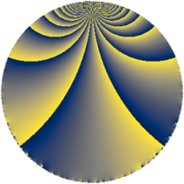Properties

 Label 1859.4.yLevel $1859$ Weight $4$ Character orbit 1859.y Rep. character $\chi_{1859}(147,\cdot)$ Character field $\Q(\zeta_{30})$ Dimension $3616$ Sturm bound $728$

Related objects

Defining parameters

 Level: $$N$$ $$=$$ $$1859 = 11 \cdot 13^{2}$$ Weight: $$k$$ $$=$$ $$4$$ Character orbit: $$[\chi]$$ $$=$$ 1859.y (of order $$30$$ and degree $$8$$) Character conductor: $$\operatorname{cond}(\chi)$$ $$=$$ $$143$$ Character field: $$\Q(\zeta_{30})$$ Sturm bound: $$728$$

Dimensions

The following table gives the dimensions of various subspaces of $$M_{4}(1859, [\chi])$$.

Total New Old
Modular forms 4480 3776 704
Cusp forms 4256 3616 640
Eisenstein series 224 160 64

Trace form

 $$3616 q + 9 q^{2} + 3 q^{3} - 1765 q^{4} + 9 q^{6} + 9 q^{7} + 3939 q^{9} + O(q^{10})$$ $$3616 q + 9 q^{2} + 3 q^{3} - 1765 q^{4} + 9 q^{6} + 9 q^{7} + 3939 q^{9} + 328 q^{10} - 150 q^{11} + 12 q^{12} - 12 q^{14} + 63 q^{15} + 6527 q^{16} - 161 q^{17} - 195 q^{19} + 333 q^{20} + 829 q^{22} - 92 q^{23} + 1347 q^{24} + 20324 q^{25} - 264 q^{27} - 147 q^{28} - 97 q^{29} + 1783 q^{30} - 1032 q^{32} - 306 q^{33} - 41 q^{35} - 13216 q^{36} + 9 q^{37} - 610 q^{38} - 1544 q^{40} - 279 q^{41} - 2443 q^{42} + 1000 q^{43} + 654 q^{45} + 657 q^{46} + 1275 q^{48} - 18963 q^{49} + 966 q^{50} + 2504 q^{51} + 1412 q^{53} - 1518 q^{54} - 1047 q^{55} + 1638 q^{56} - 2175 q^{58} - 2061 q^{59} + 423 q^{61} + 2881 q^{62} + 5754 q^{63} + 41204 q^{64} + 8126 q^{66} + 1272 q^{67} + 3032 q^{68} - 4191 q^{69} + 483 q^{71} + 15123 q^{72} + 2357 q^{74} + 2947 q^{75} - 2142 q^{76} - 5466 q^{77} - 8204 q^{79} - 4377 q^{80} + 31029 q^{81} + 3565 q^{82} - 6840 q^{84} + 5511 q^{85} - 10256 q^{87} - 8815 q^{88} + 6372 q^{89} + 8162 q^{90} + 2690 q^{92} + 12849 q^{93} - 9037 q^{94} - 3629 q^{95} + 2889 q^{97} - 11394 q^{98} + O(q^{100})$$

Decomposition of $$S_{4}^{\mathrm{new}}(1859, [\chi])$$ into newform subspaces

The newforms in this space have not yet been added to the LMFDB.

Decomposition of $$S_{4}^{\mathrm{old}}(1859, [\chi])$$ into lower level spaces

$$S_{4}^{\mathrm{old}}(1859, [\chi]) \cong$$ $$S_{4}^{\mathrm{new}}(143, [\chi])$$$$^{\oplus 2}$$